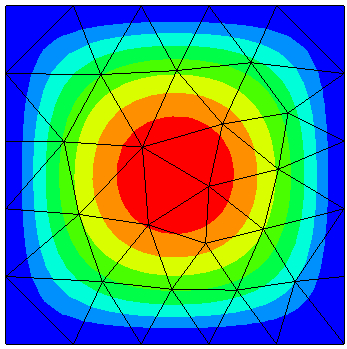# Poisson equation¶

We solve the Poisson equation on the unit-square, with homogeneous Dirichlet boundary conditions. You can run the `example` either directly within the Python interpreter (Python version 3 is required!):

```python3 poisson.py
```

or you can run it with Netgen providing you also a graphical user interface

```netgen poisson.py
```
```# solve the Poisson equation -Delta u = f
# with Dirichlet boundary condition u = 0

from ngsolve import *
from netgen.geom2d import unit_square

ngsglobals.msg_level = 1

# generate a triangular mesh of mesh-size 0.2
mesh = Mesh(unit_square.GenerateMesh(maxh=0.2))

# H1-conforming finite element space
fes = H1(mesh, order=3, dirichlet=[1,2,3,4])

# define trial- and test-functions
u = fes.TrialFunction()
v = fes.TestFunction()

# the right hand side
f = LinearForm(fes)
f += 32 * (y*(1-y)+x*(1-x)) * v * dx

# the bilinear-form
a = BilinearForm(fes, symmetric=True)

a.Assemble()
f.Assemble()

# the solution field
gfu = GridFunction(fes)
gfu.vec.data = a.mat.Inverse(fes.FreeDofs(), inverse="sparsecholesky") * f.vec
# print (u.vec)

# plot the solution (netgen-gui only)
Draw (gfu)